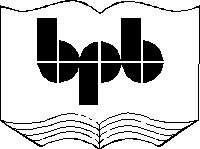5

Float library

A float is a number that has decimal places. These numbers are required for various types of applications where accuracy is of essence and, integer values alone cannot suffice. In this chapter, we shall cover some of the main functions that are provided in the Float Library. These functions are used to carry out necessary operations and manipulations on the float numbers.

As is the case of ints, the float library also has two similar functions viz. maxfloat and minfloat.

aaa.wmls

extern function abc()

{

var aa,i;

i = Float.maxFloat();

aa = String.format("%f..",i);

i = Float.minFloat();

aa = String.format("%f..",i);

}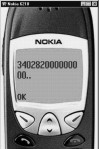Screen 5. 1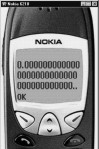Screen 5. 2

A floating-point number normally takes up 8 memory locations. It can hold a very large range of numbers. The range encompasses numbers ranging upto 10 raised to the power of 38.  This means that it can have a number that has 38 zeroes. The IEEE has a standard that talks about how floating point numbers should be represented in memory.  If you pass a floating point number to a function that expects an integer value, the number will be converted to an integer using the Float.int function.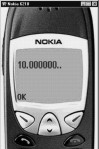Screen 5. 3

aaa.wmls

extern function abc()

{

var aa,i;

i = Float.int(10.6);

aa = String.format("%f..",i);

}

When we passed the value of 10.6 to the function, it converted it to an integer and displayed the value of i as 10. If you use the %f format specifier, the value that will be displayed is 10.000000. Thus, the decimal portion consisting of the value .6 gets removed. If you pass an integer to the Float.int function, it remains unchanged. And, if you pass a negative number, the value remains negative. Thus, the number does not change.

There is a function called Float.sqrt which can be used to find the square-root of a number. This function will be extensively required while carrying out financial or engineering calculations.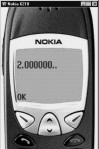Screen 5. 4

aaa.wmls

extern function abc()

{

var aa,i;

i = Float.sqrt(4);

aa = String.format("%f..",i);

}

Finding the exact square root of a floating point number is cumbersome. For example, the square root of 4 is 2, but the square root of 3 is 1.732051. By default, only the first 6 digits are displayed, but the exact square root is a much larger number. Therefore,  a square root is always an exponentiation.

A drawback of a large number of programming languages is that they don't provide an operator for exponentiation. It is generally implemented as a function. Float has a function called power, through which we can return the power of a number. For example, if we pass the parameters 3,2 to this function, it implies 3 raised to the power of 2 i.e. 9.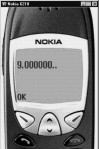Screen 5. 5

aaa.wmls

extern function abc()

{

var aa,i;

i = Float.pow(3,2);

aa = String.format("%f..",i);

}

You can pass different parameters to this function. Some of the possibilities are as follows:

· If the first parameter is 0 and the second parameter is less than 0, the answer will be invalid.

· If the first parameter is negative and the second parameter is not an integer, it is invalid.

· If the first parameter is negative then, the second parameter must be an integer.

NOTE : 3.1 raised to 2 and -3.1 raised to 2 gives the same answer.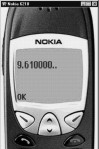Screen 5. 6

aaa.wmls

extern function abc()

{

var aa,i;

i = Float.pow(3.1,2);

aa = String.format("%f..",i);

}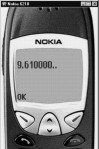Screen 5. 7

aaa.wmls

extern function abc()

{

var aa,i;

i = Float.pow(-3.1,2);

aa = String.format("%f..",i);

}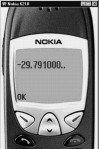Screen 5. 8

aaa.wmls

extern function abc()

{

var aa,i;

i = Float.pow(-3.1,3);

aa = String.format("%f..",i);

}

You always need a mechanism to round off a number. To achieve this, a function called round has been provided for, in the Float library.

Example : If you pass the parameter 6.4 to this function, it gets rounded off to 6 .

But if you pass the value 6.5 and above, it rounds it off to 7.Screen 5. 9

aaa.wmls

extern function abc()

{

var aa,i;

i = Float.round(6.4);

aa = String.format("%d..",i);

}

This fact makes it evident that if the decimal portion of a positive number is .5 and above, the number is rounded off to the next higher number. But if it is less than .5, it gets rounded off to the next lower number. In the case of a negative number, if the decimal portion is .5 or less, it gets rounded off to the next higher number.  For instance,  -6.5 will be rounded off to -6 because, -6 has a higher value than -6.5. If the decimal portion is .51 or more, it gets rounded off to the next lower number; for eg. -6.51 gets rounded off to -7 because it is the next lower number. The function round always returns an integer value.

Float also has the functions called ceil and floor. Ceil is the short form for ceiling. It rounds off the number to the next higher value. However, Floor rounds off the number to the next lower number.  The difference between round and the set of functions consisting of ceil and floor can be explained by an example :

If the number 1.6 is given as input to these functions, the outputs will be as follows:

· round will return a value of 2

· floor will return a value of 1

· ceil will return a value of 2 .

aaa.wmls

extern function abc()

{

var aa,i;

i = Float.floor(1.6);

aa = String.format("floor %d..",i);

i = Float.ceil(1.6);

aa = String.format("ciel %d..",i);

}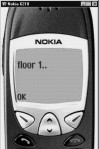Screen 5. 10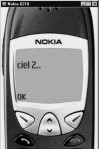Screen 5. 11

Thus, the return value of the function round depends on the value of the decimal portion of the number, whereas, ceil and floor forcibly round off the number to the next higher and lower numbers, respectively.

aaa.wmls

extern function abc()

{

var aa,i;

i = Float.floor(1.2);

aa = String.format("floor %d..",i);

i = Float.ceil(1.2);

aa = String.format("ciel %d..",i);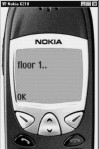Screen 5. 12Screen 5. 13